GeeksforGeeks App
Open AppBrowser
Continue

# What do you mean by jQuery traversing ?

jQuery traversing means moving through the linked elements in an HTML page. Using jQuery traversing, we can search and find different elements based on the relation of the elements. The elements can be parents, siblings, children, etc.

We can move up (ancestors), down (descendants), and sideways (siblings). We can see a DOM in the following diagram. It is just for demonstration of how DOM works and is not perfect. Starting from any element in the DOM, we can reach any element in the DOM because every element is a descendant of HTML which is part of traversing.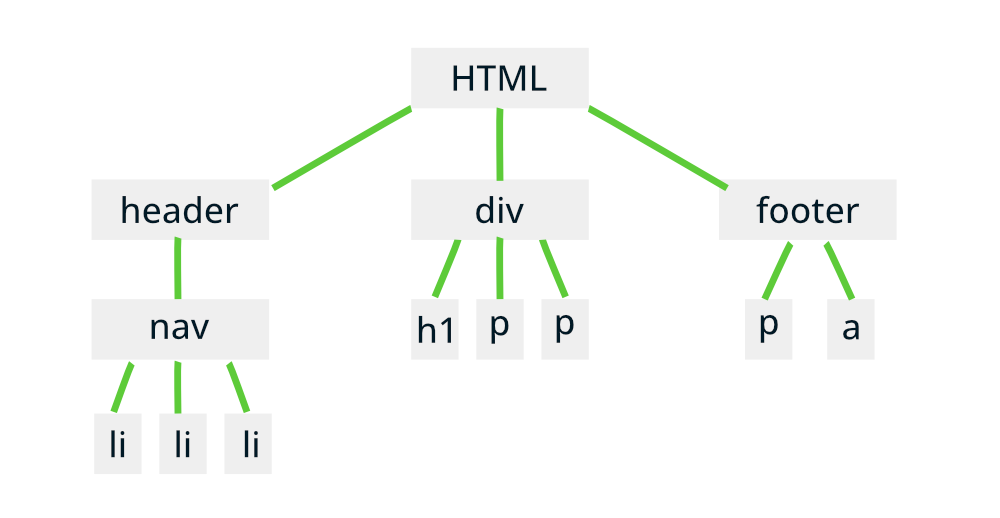DOM

The following things can be concluded from the above illustration.

• The <html> is the root element.
• The <header>, <div> and <footer> are the children of <html> element.
• The <nav> is the child of <header> which in turn is parent of three <li> elements.
• Again <div> element, sibling of <header> is ancestor of <h1> and two <p>.
• The <footer> is the successor of <html>, which is ancestor of <p> and <a>.

Traversing Up: Traversing up the DOM means moving towards the parents or ancestors. The following three methods are used to traverse up in jQuery.

jQuery parent() Method: This method is used to get the nearest parent for the element we are searching for. The following is an example where we are finding the parent of <li> which is <ul>. We used the CSS parent() function to get the direct parent. <ul> is the direct parent of both the <li> elements.

## HTML

 ```<``html``>`` ` `<``head``>``    ``<``meta` `charset``=``"utf-8"``>``    ``<``meta` `name``=``"viewport"` `content``=``"width=device-width"``>``    ``<``title``>GeeksforGeeks``    ``<``script` `src``=``"https://ajax.googleapis.com/ajax/libs/jquery/3.5.1/jquery.min.js"``>``    `````` ` `<``body``>``    ``<``h1``>GeeksforGeeks``    ``<``div``>``        ``<``h4``>Welcome to GeeksforGeeks``        ``<``ul``>``            ``<``li``>Data Structures``            ``<``li``>Algorithms``        ````    ````    ``<``script``>``        ``\$("li").parent().css({``            ``"color": "green",``            ``"margin": "2rem",``            ``"border": "2px solid green"``        ``})``    ```````

Output: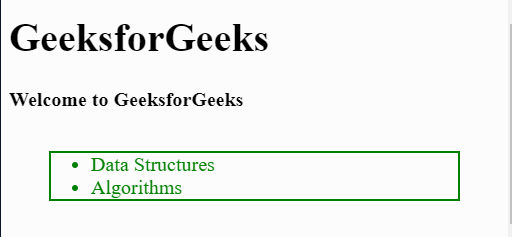jQuery parents() Method: This method is used to get all the ancestors up to the root of the document that is the <html>. In the following example, we have a nested tree of elements and we will apply a style to all the ancestors of the <li> item having the id of “lastchild”.

## HTML

 ```<``html``>`` ` `<``head``>``    ``<``meta` `charset``=``"utf-8"``>``    ``<``meta` `name``=``"viewport"` `content``=``"width=device-width"``>``    ``<``title``>GeeksforGeeks``    ``<``script` `src``=``"https://ajax.googleapis.com/ajax/libs/jquery/3.5.1/jquery.min.js"``>``    `````` ` `<``body``>``    ``<``h1``>GeeksforGeeks``    ``<``div``>``        ``<``h4``>Welcome to GeeksforGeeks``        ``<``ul``>``            ``<``li``>``                ``Data Structures``                ``<``ul``>``                    ``<``li` `id``=``"lastchild"``>Array``                    ``<``li``>Struct``                    ``<``li``>Queue``                    ``<``li``>Linked List``                ````            ````            ``<``li``>``                ``Algorithms``                ``<``ul``>``                    ``<``li``>Searching``                    ``<``li``>Sorting``                    ``<``li``>Backtracking``                ````            ````        ````    ````    ``<``script``>``        ``\$('#lastchild').parents().css({``            ``"margin": ".2rem",``            ``"border": "2px solid green"``        ``})``    ```````

Output: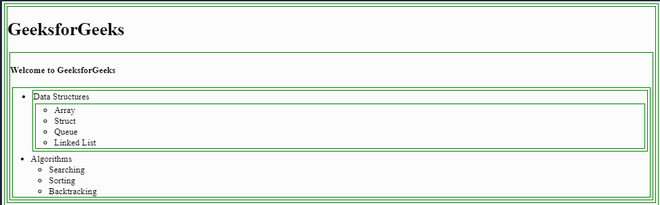jQuery parentsUntil() Method: This method of jQuery is used to get the parents that are up to the declared element only. The following example has an HTML div that has a list of items. We want the style up to the first <ul> that has the id of “finalparent” starting from the “lastchild” id of <li> item.

So the border and margin should be applied to two elements. first the direct parent of the <ul> and then the <li>

## HTML

 ```<``html``>`` ` `<``head``>``    ``<``meta` `charset``=``"utf-8"``>``    ``<``meta` `name``=``"viewport"` `content``=``"width=device-width"``>``    ``<``title``>GeeksforGeeks``    ``<``script` `src``=``"https://ajax.googleapis.com/ajax/libs/jquery/3.5.1/jquery.min.js"``>``    `````` ` `<``body``>``    ``<``h1``>GeeksforGeeks``    ``<``div``>``        ``<``h4``>Welcome to GeeksforGeeks``        ``<``ul` `id``=``"finalparent"``>``            ``<``li``>``                ``Data Structures``                ``<``ul``>``                    ``<``li` `id``=``"lastchild"``>Array``                    ``<``li``>Struct``                    ``<``li``>Queue``                    ``<``li``>Linked List``                ````            ````            ``<``li``>``                ``Algorithms``                ``<``ul``>``                    ``<``li``>Searching``                    ``<``li``>Sorting``                    ``<``li``>Backtracking``                ````            ````        ````    ````    ``<``script``>``        ``\$('#lastchild').parentsUntil("#finalparent").css({``            ``"margin": ".2rem",``            ``"border": "2px solid green"``         ``})``    ```````

Output: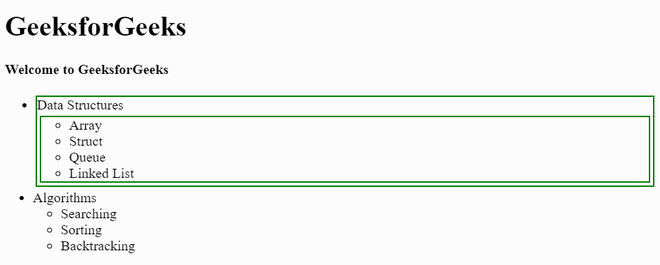Traversing Down: Traversing down means we want to reach the descendants of the ancestor elements. The descendant can be the direct child or a nested child. We can find the different descendants in jQuery using the following two functions.

jQuery children() Method: Using this jQuery function, we can access all the direct children of the element. Direct children mean the elements just inside the element and not the elements further nested.

The following is an example where the <div> element has three <p> paragraphs. The result will have all the paragraphs with a green border.

## HTML

 ```<``html``>`` ` `<``head``>``    ``<``meta` `charset``=``"utf-8"``>``    ``<``meta` `name``=``"viewport"` `content``=``"width=device-width"``>``    ``<``title``>GeeksforGeeks``    ``<``script` `src``=``"https://ajax.googleapis.com/ajax/libs/jquery/3.5.1/jquery.min.js"``>``    `````` ` `<``body``>``    ``<``h1``>GeeksforGeeks``    ``<``div``>``        ``<``p``>GeeksforGeeks is a coding and development tutorial website.``        ``<``p``>We can also find tutorials for Machine Learning and IOT.``        ``<``p``>Almost all of the resources on GeeksforGeeks is for free.`` ` `    ````    ``<``script``>``        ``\$('div').children().css({``            ``"margin": "1rem",``            ``"border": "2px dotted green"``        ``})``    ```````

Output:jQuery find() Method: In this function. we pass the name, id, or class name of the element that is the direct or indirect child of the element. In this example, we want to find the <li> item having the id of “lastchild” which is the indirect descendant of <div>.

## HTML

 ```<``html``>`` ` `<``head``>``    ``<``meta` `charset``=``"utf-8"``>``    ``<``meta` `name``=``"viewport"` `content``=``"width=device-width"``>``    ``<``title``>GeeksforGeeks``    ``<``script` `src``=``"https://ajax.googleapis.com/ajax/libs/jquery/3.5.1/jquery.min.js"``>``    `````` ` `<``body``>``    ``<``h1``>GeeksforGeeks``    ``<``div``>``        ``<``p``>``            ``GeeksforGeeks is a coding and ``            ``development tutorial website.``        ````        ``<``p``>``            ``We can also find tutorials for ``            ``Machine Learning and IOT.``        ````        ``<``p``>``            ``Almost all of the resources on ``            ``GeeksforGeeks is for free.``        ```` ` `        ``<``ul``>``            ``<``li``>Algorithms``            ``<``li` `id``=``"lastchild"``>Data Structures``            ``<``li``>Machine Learning``        ````    ````    ``<``script``>``        ``\$('div').find("#lastchild").css({``            ``"padding": ".5rem",``            ``"margin": "1rem",``            ``"border": "5px solid green"``        ``})``    `````` ` ``

Output: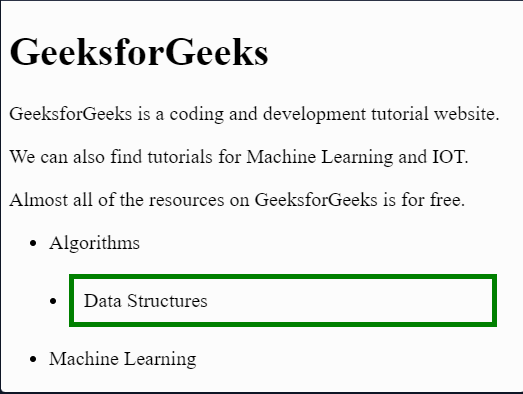Traversing Siblings: Siblings can be related to brothers and sisters. Suppose the list items of the unordered list are all siblings. We can use the following functions to find the siblings in jQuery.

jQuery siblings() Method: This method is used to find all the sideways elements where it is on top or beneath except the element itself. This method finds all the siblings of the <h3> tag and to identify the elements, a CSS is applied.

## HTML

 ```<``html``>`` ` `<``head``>``    ``<``meta` `charset``=``"utf-8"``>``    ``<``meta` `name``=``"viewport"` `content``=``"width=device-width"``>``    ``<``title``>GeeksforGeeks``    ``<``script` `src``=``"https://ajax.googleapis.com/ajax/libs/jquery/3.5.1/jquery.min.js"``>``    `````` ` `<``body``>``    ``<``h1``>GeeksforGeeks``    ``<``div``>``        ``<``h3``>Welcome to GeeksforGeeks``        ``<``p``>``            ``GeeksforGeeks is a coding and ``            ``development tutorial website.``        ````        ``<``p``>``            ``We can also find tutorials for ``            ``Machine Learning and IOT.``        ```` ` `        ``<``ul``>``            ``<``li``>Algorithms``            ``<``li``>Data Structures``            ``<``li``>Machine Learning``        ````    ```` ` `    ``<``script``>``        ``\$('h3').siblings().css({``            ``"margin": "1rem",``            ``"border": "5px solid green"``        ``})``    ```````

Output:jQuery next() Method: This method is used to find the just next sibling element. In the following example, we are finding the element next to the <h3> that is the first <p> element.

## HTML

 ```<``html``>`` ` `<``head``>``    ``<``meta` `charset``=``"utf-8"``>``    ``<``meta` `name``=``"viewport"` `content``=``"width=device-width"``>``    ``<``title``>GeeksforGeeks``    ``<``script` `src``=``"https://ajax.googleapis.com/ajax/libs/jquery/3.5.1/jquery.min.js"``>``    `````` ` `<``body``>``    ``<``h1``>GeeksforGeeks``    ``<``div``>``        ``<``h3``>Welcome to GeeksforGeeks``        ``<``p``>``            ``GeeksforGeeks is a coding and ``            ``development tutorial website.``        ```` ` `        ``<``p``>``            ``We can also find tutorials for ``            ``Machine Learning and IOT.``        ```` ` `        ``<``ul``>``            ``<``li``>Algorithms``            ``<``li``>Data Structures``            ``<``li``>Machine Learning``        ````    ````    ``<``script``>``        ``\$('h3').next().css({``            ``"margin": "1rem",``            ``"border": "5px solid green"``        ``})``    ```````

Output:jQuery nextAll() Method: This method is very similar to the next() method but it finds all the elements next to the element we want to find. In the following example, we want to find the elements next to the <p> element that has an id

## HTML

 ```<``html``>`` ` `<``head``>``    ``<``meta` `charset``=``"utf-8"``>``    ``<``meta` `name``=``"viewport"` `content``=``"width=device-width"``>``    ``<``title``>GeeksforGeeks``    ``<``script` `src``=``"https://ajax.googleapis.com/ajax/libs/jquery/3.5.1/jquery.min.js"``>``    `````` ` `<``body``>``    ``<``h1``>GeeksforGeeks``    ``<``div``>``        ``<``h3``>Welcome to GeeksforGeeks``        ``<``p``>GeeksforGeeks is a coding and development tutorial website.``        ``<``p` `id``=``"paragraph"``>``            ``We can also find tutorials for Machine Learning and IOT.``        ```` ` `        ``<``ul``>``            ``<``li``>Algorithms``            ``<``li``>Data Structures``            ``<``li``>Machine Learning``        ````    ````    ``<``script``>``        ``\$('#paragraph').nextAll().css({"margin": "1rem",``                                       ``"border": "5px solid green"})``    `````` ` ``

Output:jQuery nextUntil() Method: This method is used to find the sibling elements until the element is specified or passed to this function. In this example, we are starting from the first <p> element and we will move until the <ul>.

## HTML

 ```<``html``>`` ` `<``head``>``    ``<``meta` `charset``=``"utf-8"``>``    ``<``meta` `name``=``"viewport"` `content``=``"width=device-width"``>``    ``<``title``>GeeksforGeeks``    ``<``script` `src``=``"https://ajax.googleapis.com/ajax/libs/jquery/3.5.1/jquery.min.js"``>``    `````` ` `<``body``>``    ``<``h1``>GeeksforGeeks``    ``<``div``>``        ``<``h3``>Welcome to GeeksforGeeks``        ``<``p` `id``=``"paragraph"``>``          ``GeeksforGeeks is a coding and ``          ``development tutorial website.``        ```` ` `        ``<``p``>We can also find tutorials for Machine Learning and IOT.`` ` `        ``<``ul``>``            ``<``li``>Algorithms``            ``<``li``>Data Structures``            ``<``li``>Machine Learning``        ````    ````    ``<``script``>``        ``\$('#paragraph').nextUntil("ul").css({"margin": "1rem",``                                             ``"border": "5px solid green"})``    `````` ` ``

Output:jQuery prev() Method: The prev() function finds the element that is before or above the current element. Both the elements are siblings. It finds the previous element to it. So we used this function in the following example to find the previous element to the <p> element that has an id.

## HTML

 ```<``html``>`` ` `<``head``>``    ``<``meta` `charset``=``"utf-8"``>``    ``<``meta` `name``=``"viewport"` `content``=``"width=device-width"``>``    ``<``title``>GeeksforGeeks``    ``<``script` `src``=``"https://ajax.googleapis.com/ajax/libs/jquery/3.5.1/jquery.min.js"``>``    `````` ` `<``body``>``    ``<``h1``>GeeksforGeeks``    ``<``div``>``        ``<``h3``>Welcome to GeeksforGeeks``        ``<``p` `id``=``"paragraph"``>``            ``GeeksforGeeks is a coding and ``            ``development tutorial website.``        ```` ` `        ``<``p``>``            ``We can also find tutorials for ``            ``Machine Learning and IOT.``        ```` ` `        ``<``ul``>``            ``<``li``>Algorithms``            ``<``li``>Data Structures``            ``<``li``>Machine Learning``        ````    ````    ``<``script``>``        ``\$('#paragraph').prev().css({"margin": "1rem",``                                    ``"border": "5px solid green"})``    `````` ` ``

Output:jQuery prevAll() Method: This function is used to find all the elements that are siblings and previous to the current element. This method is similar to the nextAll() function. In the following example, we are finding the elements before <ul> element.

## HTML

 ```<``html``>`` ` `<``head``>``    ``<``meta` `charset``=``"utf-8"``>``    ``<``meta` `name``=``"viewport"` `content``=``"width=device-width"``>``    ``<``title``>GeeksforGeeks``    ``<``script` `src``=``"https://ajax.googleapis.com/ajax/libs/jquery/3.5.1/jquery.min.js"``>``    `````` ` `<``body``>``    ``<``h1``>GeeksforGeeks``    ``<``div``>``        ``<``h3``>Welcome to GeeksforGeeks``        ``<``p``>``            ``GeeksforGeeks is a coding and ``            ``development tutorial website.``        ````        ``<``p``>``            ``We can also find tutorials for ``            ``Machine Learning and IOT.``        ```` ` `        ``<``ul``>``            ``<``li``>Algorithms``            ``<``li``>Data Structures``            ``<``li``>Machine Learning``        ````    ````    ``<``script``>``        ``\$('ul').prevAll().css({"margin": "1rem",``                               ``"border": "5px solid green"})``    `````` ` ``jQuery prevUntil() Method: This function finds all the elements previous to the current element until the specified element. In the following example, we want to find all previous elements of <ul> until the <h3> element. The <h3> will not be included.

## HTML

 ```<``html``>`` ` `<``head``>``    ``<``meta` `charset``=``"utf-8"``>``    ``<``meta` `name``=``"viewport"` `content``=``"width=device-width"``>``    ``<``title``>GeeksforGeeks``    ``<``script` `src``=``"https://ajax.googleapis.com/ajax/libs/jquery/3.5.1/jquery.min.js"``>``    `````` ` `<``body``>``    ``<``h1``>GeeksforGeeks``    ``<``div``>``        ``<``h3``>Welcome to GeeksforGeeks``        ``<``p``>``            ``GeeksforGeeks is a coding and ``            ``development tutorial website.``        ````        ``<``p``>``            ``We can also find tutorials for ``            ``Machine Learning and IOT.``        ```` ` `        ``<``ul``>``            ``<``li``>Algorithms``            ``<``li``>Data Structures``            ``<``li``>Machine Learning``        ````    ````    ``<``script``>``        ``\$('ul').prevUntil('h3').css({"margin": "1rem",``                                     ``"border": "5px solid green"})``    `````` ` ``

Output:My Personal Notes arrow_drop_up
Related Tutorials